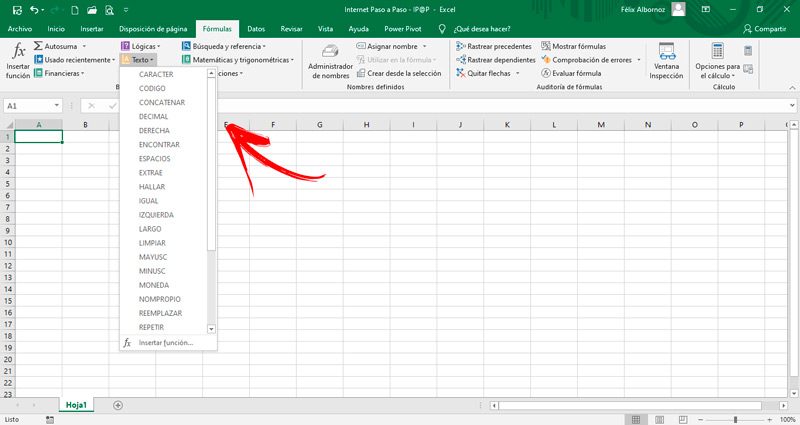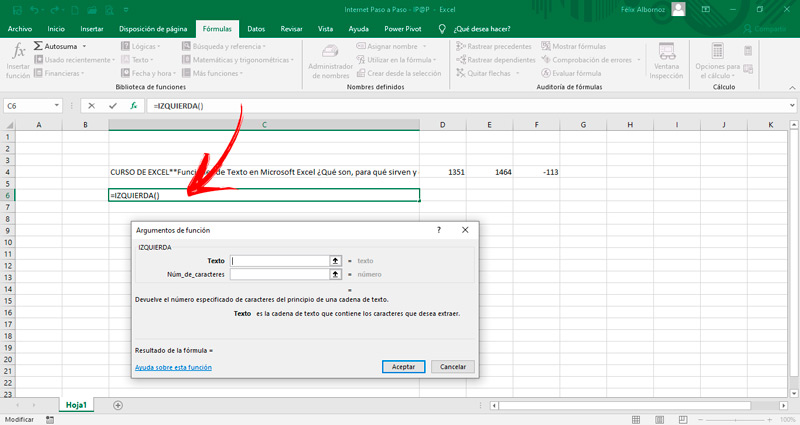Excel Text Functions  What are they? + List ▷ 2020

When you work with files that you migrate from other programs and need to convert them into text formats so that you can find words, convert them from uppercase to lowercase or vice versa, you will need to handle Excel text formulas.

In this way you will be able to become an expert because you will save a lot of time and you will not make mistakes, since you will find the results in a practical and fast way.

We will show you below, all the text functions that Microsoft Excel provides to users. You will be able to find an example of each one so that you can put it into practice in a simple way.

What is a text formula in Microsoft Excel and what are they for in spreadsheets?

The text formulas are tools used in Excel to convert numbers and other types of arguments into words. In this way, you can use a spreadsheet to extract words, find certain characters, or replace one sentence with another.

They are very useful functions that can be used in a simple way, You just have to pay attention and get to know them all so that you can find the one that best suits your needs.

List of all text functions available in Excel and how to use them without errorsThe text functions that you can use in Excel are the following:

• CHARACTER. This formula allows you to write using the code numbers that the operating system has. If you write CHARACTER (97) the result will be the letter a.
• CODE. When you want to get the code for the first character, you will have to use this formula. This is related to the previous function, since if you do not know the number you will have to use this tool first.
• CONCATENATE. In case you want to have a text with several elements in a single cell, you will have to use this function. It is ideal to apply it in programs where you have different columns and you want to put all that data so that the other software can recognize it. To use it, you will have to choose all the data and then press Enter.
• DECIMAL. When you have a number and you want to convert it to text, you must first round it up. For this you will have to choose the number, establish the decimals and then choose true or false in case there are no commas. For example, DECIMAL (145.678, 2, TRUE) = 145.68
• RIGHT. If you have a text string and you want to extract the characters that are located on the right, you will have to use this formula to make the last word stand out. You can also include the number of characters you need to get. You should use it this way: RIGHT (“Internet Step by Step”, 4) = “Step”> RIGHT (“Internet Step by Step”) = “o”
• FIND. When you need to find a certain text, you can find the starting position within a text string. You can do this like this: FIND («I», «IPAP») = 1; FIND (“A”, “IPAP”) = 3
• SPACES. This function is useful when you need to remove the spaces that exist within a text, with the exception of the individual spaces that the words have. An example of this is: SPACES (»Internet«) = «Internet»; SPACES (»IPAP») = «IPAP»
• EXTRACT. You can find the number of characters in a text string by setting the position of the first letter and then telling the program the characters to extract from the indicated position. For example: EXTRAE («InternetPasoaStep», 9, 6) = «PasoaP»; EXTRAE (“InternetPasoaPaso”, 14, 4) = “Paso”
• FIND. If you need to know the position of a character within a text string, you will have to use this song. You will have to indicate the text string in which you want to find the word and indicate If you want to establish the initial position of where the search should start. If this data is omitted, Excel considers that it will start at 1. The correct use is: FIND (“Internet”, “InternetPasoaPaso”) = 1; FIND (“Step”, “InternetPasoaPaso”) = 9
• SAME. It is one of the most useful functions that you can find in the text group. Compare if two text strings are equal by returning the value TRUE or FALSE. For this it is necessary to incorporate the two chains. Its use is: EQUAL (“InternetPasoaPaso”, “InternetPasoaPaso”) = TRUE; SAME (“InternetStep”, “internetStep”) = FALSE
• LEFT. As with the RIGHT function, this tool returns the character values ​​that are to the left of a text string. For example, it should be used like this: LEFT (“Internet Step by Step”, 8) = “Internet”; LEFT («Internet Step by Step») = «I»
• LONG. You will be able to know the number of characters that a certain text has. You will have to use it like this: LONG (“Internet”) = 8; LONG (“IPAP”) = 4
• CLEAN. In case you need to remove the characters that cannot be printed, you will have to use this tool. An example of this would be: CLEAN (CHARACTER (17) & «Internet» & CHARACTER (25)) = «Internet»
• MAYUSC. This function converts a text string into uppercase letters. For example, UPPERCASE (“Internet Step by Step”) = “INTERNET STEP BY STEP”; UPPER (“ipap”) = “IPAP”
• MINUSC. As with the previous function, with this tool you can convert texts into lowercase letters. MINUSC (“INTERNET STEP BY STEP”) = “internet step by step”; MINUSC (“Internet Step by Step”) = “internet step by step”
• COIN. If you are looking to convert a number into text format you will have to use this function. In addition, it will incorporate the currency sign. You will have the possibility to include the number of decimal places that you want to appear in the result. The use that you must make of this function is as follows: CURRENCY (1203) = «1203.00 €»; CURRENCY (24.858, 2) = «24.86 €»
• NOMPROPIO. You can convert a string of texts into uppercase and lowercase with the first letter of each word being uppercase. An example for this use is the following NOMPROPIO (“internet step by step”) = “Internet step by step”; NOMPROPIO (“microsoft spreadsheet program”) = “Microsoft spreadsheet program”
• REPLACE. In case you need to replace a text string with another you will have to use this function. You will have to incorporate the original text (which contains the characters that you need to change), the position it will have within that text string and where the replacement task will have to start from, the amount of characters you want to replace and the text that will be the one that replaces the original characters. REPLACE (“0123456789”, 3, 6, “IPAP”) = “01IPAP89”; REPLACE (“Year 2019”, 6, 2, “20”) = “Year 2020”
• REPEAT. You can repeat a text as many times as you need with this tool. For this you will have to choose the text and indicate how many times it will be repeated. For example: REPEAT (“Internet”, 3) = “InternetInternetInternet”; REPEAT (“IPAP”, 2) = “IPAPIPAP”
• REPLACE. When you use this function you will be able to replace one text with another. You will need to establish the text that will make the change, you will have to indicate the text in which the modification will be made, the text it will be replaced with and, if you wish, the appearance it will have each time it is replaced. An example of this usage is: SUBSTITUTE (“123456”, “56”, “5678”) = “12345678”; SUBSTITUTE (“InternetInternetInternet”, “Internet”, “IPAP”, 2) = “InternetIPAPInternet”
• T. This function is used when you have a large amount of data and you need to know if a value is text. In case it is not, return a result in quotes. For example it should be used like this: T (“Internet_Step_a_Step”) = “Internet_Step_a_Step”; T (766.98) = «»
• TEXT. You can convert a value to text using a specific number format. You will need to include the numeric value that must then be converted to a text function and then in the format to which you want to apply that amount. An example of this is: TEXT (446, «0.00 €») = «446.00 €»
• TEXTBAHT. You can convert a number to text from the Thai unit of measure. An example: TEXTOBAHT (443) = สอง ร้อย สามสิบ สาม บาท ถ้วน. That is, it shows number 443 in a text from that country.
• UNICAR. With this function you can find the UNICODE code that corresponds to a specific number. For example, UNICAR (“82”) = “R”
• UNICODE. This function will let you know the unique code of the first letter of a text. The correct use of this formula will be UNICODE (“InternetPasoaPaso”) = “73
• VALUE. When you export numbers from a table from other software or on the Internet, they are converted into texts, presented as numbers. To be able to add or perform any arithmetic operation you will have to convert them into numbers. For this you will have to use this formula: VALUE («248») = 248; VALUE (“internet”) = # VALUE!
• VALUE.NUMBER. This function can be used to convert a text to a number independently of the regional settings you have on your computer. You must bear in mind that there are countries where the comma is used to start writing the units of a thousand and to the point they use it to separate the cents. Examples of the use of this tool are: VALUE.NUMBER (“1,600.77”. “.”. “,”) = 1600.77; VALUE.NUMBER (“7.8%”) = 0.078If you have any questions, leave them in the comments, we will answer you as soon as possible, and it will also be of great help to more members of the community. Thank you! 😉

Rate this post